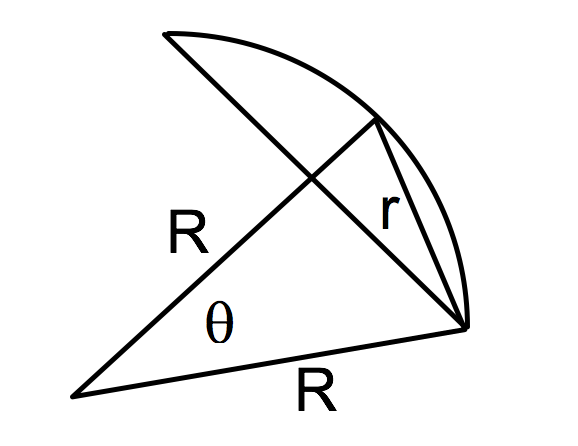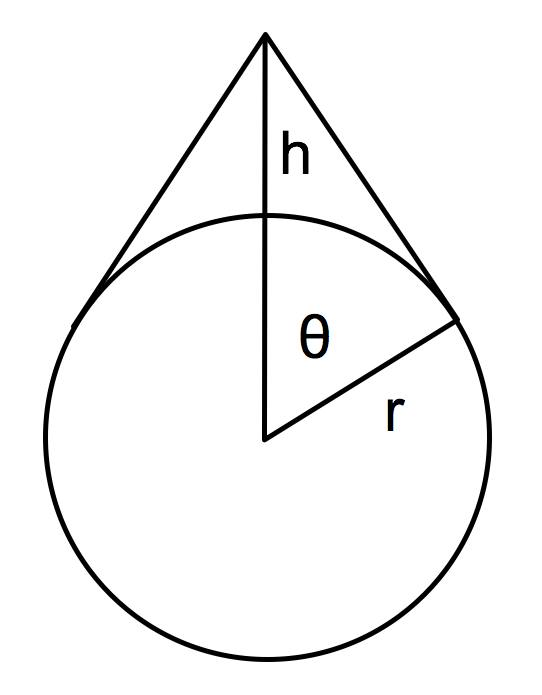## ________________

With the new year approaching, the passage of time is on people’s minds.

## ________________### Breakfast, Lunch, or Dinner?

Just as team member Malcolm is finishing a meal, he notices the minute hand is on a minute mark exactly one minute ahead of the hour hand.

Which meal is Malcolm finishing?

### The 2018 Puzzle

The coach has an interesting problem to celebrate the coming year.

“You have 2018 distinct points in space. Prove that there is a plane that divides the points into two sets, with 1009 points on each side of the plane.”

## Solutions to week 119

In Solar Cap Versus Polar Cap, the solar cap and polar cap have equal areas. The pole in How Tall Is the Pole is ≈ 180.75 feet tall.

Solar Cap Versus Polar Cap answer explained:Let the compass radius be r. On a sphere of radius R the area of a spherical cap of half-angle θ is 2π(1 – cosθ)R2 (θ is shown in the figure.) Also from the figure, cosθ = 1 – 2sin2(θ/2) = 1 – 2r2/(2R)2. Putting these together gives the area of the spherical cap as πr2, independent of R.

How Tall Is the Pole answer explained:Let h be the height of the pole. r = 20996000 feet is the earth’s radius of curvature, and d is the extra length of chain needed to accommodate the pole. Then, from the figure,
d = 2r(tanθ – θ) = 2r(θ3/3 + 2θ5/15 + …) ≈ 2rθ3/3
h = r(secθ – 1) ≈ rθ2/2 = (9d2r/32)1/3 ≈ 180.749… feet.

Another way to solve the problem is to use elementary trigonometry and the trigonometric equation solver found here: http://www.wolframalpha.com/widgets/view.jsp?id=27b8624af4f632acc36d65c4a7eb715.

## Recent Weeks

Links to all of the puzzles and solutions are on the Complete Varsity Math page.

Come back next week for answers and more puzzles.